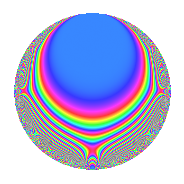# Properties

 Label 23.2.cLevel 23 Weight 2 Character orbit c Rep. character $$\chi_{23}(2,\cdot)$$ Character field $$\Q(\zeta_{11})$$ Dimension 10 Newforms 1 Sturm bound 4 Trace bound 0

# Related objects

## Defining parameters

 Level: $$N$$ = $$23$$ Weight: $$k$$ = $$2$$ Character orbit: $$[\chi]$$ = 23.c (of order $$11$$ and degree $$10$$) Character conductor: $$\operatorname{cond}(\chi)$$ = $$23$$ Character field: $$\Q(\zeta_{11})$$ Newforms: $$1$$ Sturm bound: $$4$$ Trace bound: $$0$$

## Dimensions

The following table gives the dimensions of various subspaces of $$M_{2}(23, [\chi])$$.

Total New Old
Modular forms 30 30 0
Cusp forms 10 10 0
Eisenstein series 20 20 0

## Trace form

 $$10q$$ $$\mathstrut -\mathstrut 7q^{2}$$ $$\mathstrut -\mathstrut 7q^{3}$$ $$\mathstrut -\mathstrut 3q^{4}$$ $$\mathstrut -\mathstrut 3q^{5}$$ $$\mathstrut +\mathstrut 6q^{6}$$ $$\mathstrut -\mathstrut 5q^{7}$$ $$\mathstrut +\mathstrut 4q^{8}$$ $$\mathstrut -\mathstrut 2q^{9}$$ $$\mathstrut +\mathstrut O(q^{10})$$ $$10q$$ $$\mathstrut -\mathstrut 7q^{2}$$ $$\mathstrut -\mathstrut 7q^{3}$$ $$\mathstrut -\mathstrut 3q^{4}$$ $$\mathstrut -\mathstrut 3q^{5}$$ $$\mathstrut +\mathstrut 6q^{6}$$ $$\mathstrut -\mathstrut 5q^{7}$$ $$\mathstrut +\mathstrut 4q^{8}$$ $$\mathstrut -\mathstrut 2q^{9}$$ $$\mathstrut +\mathstrut q^{10}$$ $$\mathstrut +\mathstrut 7q^{11}$$ $$\mathstrut +\mathstrut 12q^{12}$$ $$\mathstrut -\mathstrut 3q^{13}$$ $$\mathstrut +\mathstrut 9q^{14}$$ $$\mathstrut +\mathstrut 12q^{15}$$ $$\mathstrut +\mathstrut q^{16}$$ $$\mathstrut -\mathstrut 10q^{17}$$ $$\mathstrut -\mathstrut 14q^{18}$$ $$\mathstrut +\mathstrut 2q^{19}$$ $$\mathstrut -\mathstrut 9q^{20}$$ $$\mathstrut -\mathstrut 2q^{21}$$ $$\mathstrut -\mathstrut 6q^{22}$$ $$\mathstrut -\mathstrut 12q^{23}$$ $$\mathstrut -\mathstrut 38q^{24}$$ $$\mathstrut -\mathstrut 4q^{25}$$ $$\mathstrut +\mathstrut 12q^{26}$$ $$\mathstrut -\mathstrut 4q^{27}$$ $$\mathstrut +\mathstrut 7q^{28}$$ $$\mathstrut +\mathstrut 14q^{29}$$ $$\mathstrut +\mathstrut 7q^{30}$$ $$\mathstrut +\mathstrut 10q^{31}$$ $$\mathstrut +\mathstrut 21q^{32}$$ $$\mathstrut +\mathstrut 16q^{33}$$ $$\mathstrut +\mathstrut 29q^{34}$$ $$\mathstrut +\mathstrut 7q^{35}$$ $$\mathstrut +\mathstrut 27q^{36}$$ $$\mathstrut -\mathstrut 19q^{37}$$ $$\mathstrut -\mathstrut 8q^{38}$$ $$\mathstrut +\mathstrut q^{39}$$ $$\mathstrut +\mathstrut q^{40}$$ $$\mathstrut +\mathstrut 7q^{41}$$ $$\mathstrut -\mathstrut 25q^{42}$$ $$\mathstrut -\mathstrut 11q^{43}$$ $$\mathstrut -\mathstrut 34q^{44}$$ $$\mathstrut -\mathstrut 6q^{45}$$ $$\mathstrut -\mathstrut 29q^{46}$$ $$\mathstrut -\mathstrut 18q^{47}$$ $$\mathstrut +\mathstrut 18q^{48}$$ $$\mathstrut -\mathstrut 18q^{49}$$ $$\mathstrut +\mathstrut 16q^{50}$$ $$\mathstrut +\mathstrut 7q^{51}$$ $$\mathstrut -\mathstrut 20q^{52}$$ $$\mathstrut +\mathstrut 29q^{53}$$ $$\mathstrut -\mathstrut 6q^{54}$$ $$\mathstrut -\mathstrut q^{55}$$ $$\mathstrut -\mathstrut 2q^{56}$$ $$\mathstrut -\mathstrut 8q^{57}$$ $$\mathstrut -\mathstrut 23q^{58}$$ $$\mathstrut -\mathstrut 21q^{59}$$ $$\mathstrut +\mathstrut 25q^{60}$$ $$\mathstrut +\mathstrut 3q^{61}$$ $$\mathstrut +\mathstrut 4q^{62}$$ $$\mathstrut +\mathstrut 34q^{63}$$ $$\mathstrut +\mathstrut 24q^{64}$$ $$\mathstrut +\mathstrut 2q^{65}$$ $$\mathstrut +\mathstrut 2q^{66}$$ $$\mathstrut +\mathstrut 45q^{67}$$ $$\mathstrut -\mathstrut 30q^{68}$$ $$\mathstrut +\mathstrut 26q^{69}$$ $$\mathstrut +\mathstrut 38q^{70}$$ $$\mathstrut -\mathstrut 14q^{71}$$ $$\mathstrut +\mathstrut 19q^{72}$$ $$\mathstrut +\mathstrut 19q^{73}$$ $$\mathstrut +\mathstrut 10q^{74}$$ $$\mathstrut -\mathstrut 28q^{75}$$ $$\mathstrut -\mathstrut 16q^{76}$$ $$\mathstrut +\mathstrut 2q^{77}$$ $$\mathstrut -\mathstrut 4q^{78}$$ $$\mathstrut -\mathstrut 15q^{79}$$ $$\mathstrut -\mathstrut 52q^{80}$$ $$\mathstrut -\mathstrut 44q^{81}$$ $$\mathstrut +\mathstrut 16q^{82}$$ $$\mathstrut +\mathstrut 18q^{83}$$ $$\mathstrut -\mathstrut 17q^{84}$$ $$\mathstrut -\mathstrut 19q^{85}$$ $$\mathstrut -\mathstrut 11q^{86}$$ $$\mathstrut -\mathstrut 23q^{87}$$ $$\mathstrut +\mathstrut 27q^{88}$$ $$\mathstrut +\mathstrut 25q^{89}$$ $$\mathstrut -\mathstrut 20q^{90}$$ $$\mathstrut -\mathstrut 4q^{91}$$ $$\mathstrut +\mathstrut 52q^{92}$$ $$\mathstrut +\mathstrut 4q^{93}$$ $$\mathstrut +\mathstrut 17q^{94}$$ $$\mathstrut +\mathstrut 6q^{95}$$ $$\mathstrut -\mathstrut 51q^{96}$$ $$\mathstrut -\mathstrut 34q^{97}$$ $$\mathstrut +\mathstrut 17q^{98}$$ $$\mathstrut -\mathstrut 30q^{99}$$ $$\mathstrut +\mathstrut O(q^{100})$$

## Decomposition of $$S_{2}^{\mathrm{new}}(23, [\chi])$$ into irreducible Hecke orbits

Label Dim. $$A$$ Field CM Traces $q$-expansion
$$a_2$$ $$a_3$$ $$a_5$$ $$a_7$$
23.2.c.a $$10$$ $$0.184$$ $$\Q(\zeta_{22})$$ None $$-7$$ $$-7$$ $$-3$$ $$-5$$ $$q+(-\zeta_{22}+\zeta_{22}^{4}-\zeta_{22}^{5}+\zeta_{22}^{6}+\cdots)q^{2}+\cdots$$